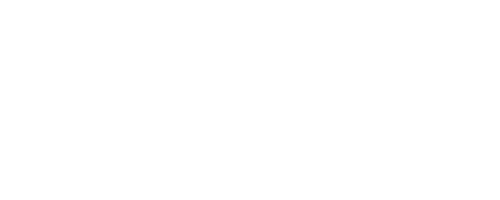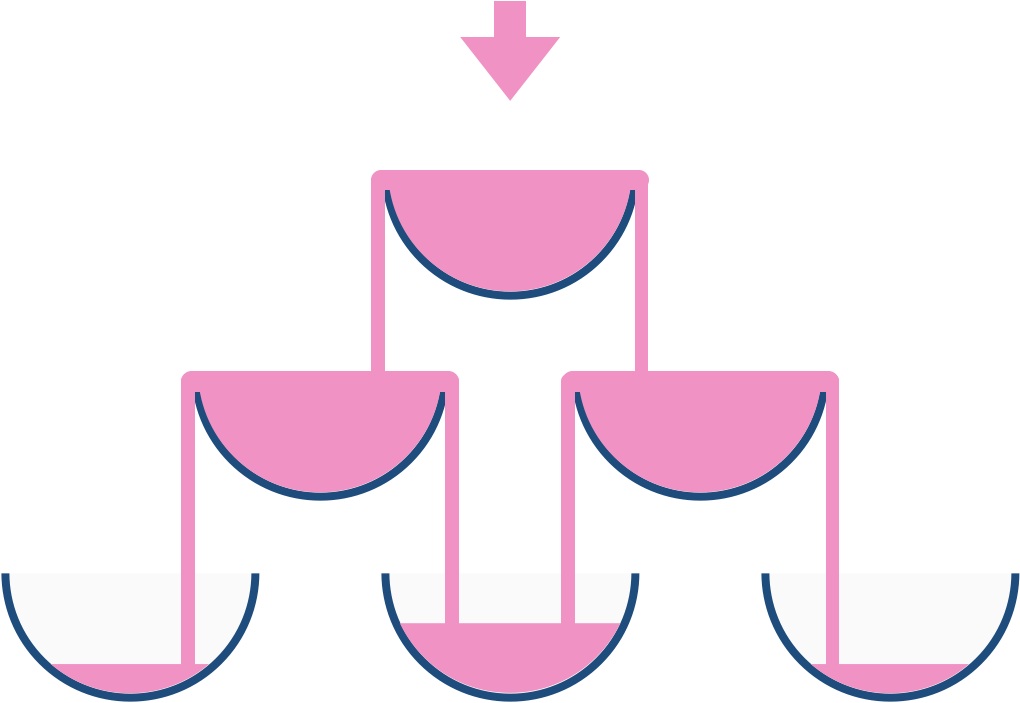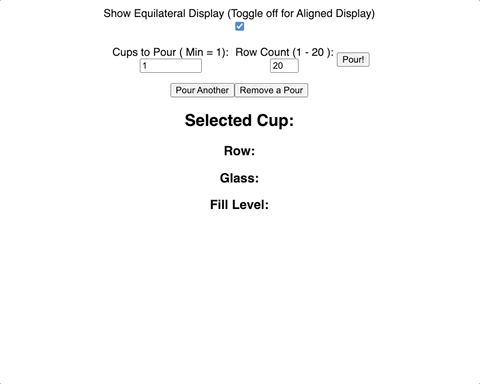<Daniel Sasse/># Algorithm Tutorial: Champagne Tower Explanation

algorithms

javascript

leetcode

codenewbie

Published: Wed May 26 2021 (~ 5 min)

Lately I have been reviewing and practicing with data structures and algorithms. I decided to begin a short series of solution walkthroughs for interesting problems I've encountered to supplement my normal tutorial guides.

Today, let's walk through the cascading champagne tower problem from Leetcode (#799)

## Contents

### Problem Description

The direct problem description found on Leetcode is:

We stack glasses in a pyramid, where the first row has 1 glass, the second row has 2 glasses, and so on until the 100th row. Each glass holds one cup of champagne.

Then, some champagne is poured into the first glass at the top. When the topmost glass is full, any excess liquid poured will fall equally to the glass immediately to the left and right of it. When those glasses become full, any excess champagne will fall equally to the left and right of those glasses, and so on. (A glass at the bottom row has its excess champagne fall on the floor.)

For example, after one cup of champagne is poured, the top most glass is full. After two cups of champagne are poured, the two glasses on the second row are half full. After three cups of champagne are poured, those two cups become full - there are 3 full glasses total now. After four cups of champagne are poured, the third row has the middle glass half full, and the two outside glasses are a quarter full, as pictured below.Essentially, this problem is modeling a waterfall cascade and is a variant of Pascal's Triangle in which each item in the Triangle is the sum of its "left parent" and "right parent". Here instead of the total sum, we need to calculate the total overflow sum.

### Problem Explanation

In reading through the problem description, we can get a sense of the cascade effect, and how the rows at the top of the tower affect those below it. However, given the row/column nature of the description, we should begin to think of the "champagne tower" as an array of arrays, where each index in the tower consists of an array whose length is one greater than the previous index: Ex: `tower = [ , [0,0], [0,0,0], ... ]`

With this in mind, instead of picturing the tower as an equilateral triangle as shown in the diagram, lets re-envision the tower so that the indexes of each row are aligned (a right triangle) and see how their values relate to each other for the same first 4 pours described in the description.

``````One Pour:
[ 1 ],
[ 0, 0 ],
[ 0, 0, 0 ],
[ 0, 0, 0, 0 ],

Two Pours:
[ 1 ],
[ 0.5, 0.5 ],
[ 0  , 0  , 0 ],
[ 0  , 0  , 0  , 0 ]

Three Pours:
[ 1 ],
[ 1  , 1 ],
[ 0  , 0  , 0 ],
[ 0  , 0  , 0  , 0 ]

Four Pours:
[ 1 ],
[ 1   , 1 ],
[ 0.25, 0.5 , 0.25 ],
[ 0   , 0   , 0   , 0 ]
``````

If we look carefully at how the indexes of the "children" glasses for an overflowing "parent", we can see that one of the recipient children has the same index and the other child is always one greater than the current index. This relationship will help us in the solution to determine where the "overflow" amount will be assigned to.

The second key thing to takeaway, as mentioned earlier, is that the children receive the sum of the overflow amount (unlike Pascal's triangle which is the full sum) and this sum cannot exceed 1 or it too will overflow. This means that for each glass, we will need to compare how much liquid is poured into the cup (directly or via overflow) to how much can remain in the glass (1) to determine the overflow amount.

With these ideas in mind, let's write a function that constructs the tower for a given number of pours and rows. This is not the final solution, or what the problem is ultimately asking for but I feel like it helps to visualize what is happening.

#### Modeling the Tower:

This function will output the nested arrays that make up the entire tower up to the row number specified, with the amounts in each glass for the given number of pours. The comments within the function will explain each step in the process. I also built a CodeSandbox visualizer for this model to help with understanding how the glasses/rows relate``````const champagneFullTower = (poured, query_row) => {
// Initialize the tower as a nested array representing the first cup.
// This first cup is where all of the liquid poured initially goes.
const tower = [[poured]]

// Iterate for each row of the tower that we are modeling.
// Access the current row, and initialize a new array for the next row
for (let i = 0; i < query_row; i++){
const currentRow = tower[i]
const nextRow = []

/*
Iterate through each cup in the current row, calculating its fill and overflow.
Its fill amount cannot exceed 1, so Math.min() will check for this.
Calculate the overflow amount by subtracting 1 from the amount available.
Overflow amount canot be negative, so Math.max() is used to ensure this.
*/
for (let j = 0; j < currentRow.length; j++){
const fillAmount = Math.min(1, currentRow[j])
const overflowAmount = Math.max(0, currentRow[j] - 1)
/*
The two "child cups" each receive 1/2 of the overflow amount.
This should accumulate with any amount it received from a different parent.
|| operator is used to handle the initial undefined state of each index.

Remember, each parent overflows to the same index below it, and index + 1
*/
nextRow[j] = nextRow[j] + overflowAmount / 2 || overflowAmount / 2
nextRow[j+1] = nextRow[j+1] + overflowAmount / 2 || overflowAmount / 2
currentRow[j] = fillAmount
}
// Add the row we just constructed to the tower
tower.push(nextRow)
}
// Return the portion of the tower we processed
}``````

### Solution

For the problem we are solving, we do not want to return the entire tower. Instead it is asking us to return the amount present in a given row or column. One way we could do this by modifying our return statement to return just the desired glass, ensuring that the maximum value returned is 1 (since we did not calculate overflows for the last row). We will also need to add in the `query_glass` parameter from Leetcode to identify the correct glass. This functionality is also modeled on the visualizer by clicking on any of the glasses.

``````const champagneTower = (poured, query_row, query_glass) => {
const tower = [[poured]]
for (let i = 0; i < query_row; i++){
const currentRow = tower[i]
const nextRow = []

for (let j = 0; j < currentRow.length; j++){
const fillAmount = Math.min(1, currentRow[j])
const overflowAmount = Math.max(0, currentRow[j] - 1)
nextRow[j] = nextRow[j] + overflowAmount / 2 || overflowAmount / 2
nextRow[j+1] = nextRow[j+1] + overflowAmount / 2 || overflowAmount / 2
currentRow[j] = fillAmount
}
tower.push(nextRow)
}
// Math.min() ensures 1 is the highest returned value
return Math.min(1, tower[query_row][query_glass])
}``````

Since we don't actually need to keep track of the entire tower to solve the problem, we could simplify the function a bit by only keeping track of the `currentRow` and `nextRow`:

``````const champagneTower = (poured, query_row, query_glass) => {
currentRow = [poured]
for (let i = 0; i < query_row; i++){
const nextRow = []
for (let j = 0; j < currentRow.length; j++){
const overflowAmount = Math.max(0, currentRow[j] - 1)
nextRow[j] = nextRow[j] + overflowAmount / 2 || overflowAmount / 2
nextRow[j+1] = nextRow[j+1] + overflowAmount / 2 || overflowAmount / 2
}
currentRow = nextRow
}
return Math.min(1, currentRow[query_glass])
}``````